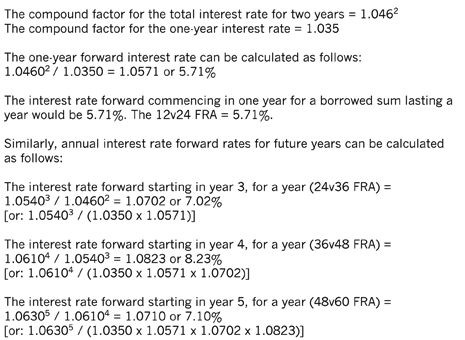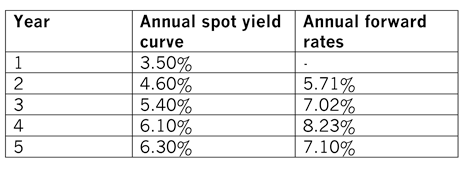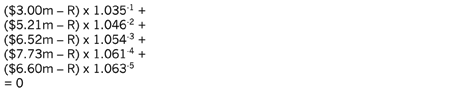# Determining interest rate forwards and their application to swap valuation

The previous article on bonds (see 'Related links') considered the relationship between bond prices, the yield curve and the yield to maturity. It demonstrated how bonds can be valued and how a yield curve may be derived using bonds of the same risk class but of different maturities. It also showed how individual company yield curves maybe estimated.

This article follows on from that, and will show how interest rate forwards may be determined from the spot yield curve. It will then briefly discuss what they mean, before proceeding to show how they may be used in determining the value of an interest rate swap. The second article addresses the learning required in the E1 and E3 areas of the Advanced Financial Management Syllabus and Study Guide.

### Determination of interest rate forwards

Supposing that a bank assesses and quotes the following rates to a company, based on the annual spot yield curve for that company’s risk class:

One-year: 3.50%
Two-year: 4.60%
Three-year: 5.40%
Four-year: 6.10%
Five-year: 6.30%

This indicates that the company would have to: pay interest at 3.50% if it wants to borrow a sum of money for one year; pay interest at 4.60% per year if it wants to borrow a sum of money for two years; pay interest at 5.40% per year if it wants borrow a sum of money for three years; and so on.

Alternatively, for a two-year loan, the company could opt to borrow a sum of money for only one year, at an interest rate of 3.50%, and then again for another year, commencing in one year’s time, instead of borrowing the money for a total of two years.

Although the company would be uncertain about the interest rate in one year’s time, it could request a forward rate from the bank that is fixed today – for example, through a 12v24 forward rate agreement (FRA). The question then arises: how may the value of the 12v24 FRA be determined?

A forward rate commencing in one year for a borrowed sum lasting a year can be calculated as follows:In summary:Supposing the company wants to borrow a sum of money for three years on the basis of the above rates:
i. it could pay annual interest at a rate of 5.40% in each of the three years, or
ii. it could pay interest at a rate 3.50% in the first year, 5.71% in the second year and 7.02% in the third year, or
iii. it could pay annual interest at a rate of 4.60% in each of the first two years and 7.02% in the third year.

### Using interest rate forwards to value a simple interest rate swap contract

Supposing the above company has \$100m borrowings in the form of variable interest rate loans repayable in five years and pays interest based on the above yield curve. It expects interest rates to increase in the future and is therefore keen to fix its interest rate payments.

The bank offers to swap the variable interest rate payments for a fixed rate, such that the company pays a fixed rate of interest to the bank in exchange for receiving a variable rate of return from the bank based on the above yield rates less 50 basis points. The variable rate receipts from the bank will then be used to pay the interest on the loan.

The fixed equivalent rate of interest the company will pay the bank for the swap can be calculated as follows:

The current expected amounts of interest the company expects to receive from the bank, based on year 1 spot rate and years 2, 3, 4 and 5 forward rates are:

Year 1     0.0300 x \$100m = \$3.00m
Year 2     0.0521 x \$100m = \$5.21m
Year 3     0.0652 x \$100m = \$6.52m
Year 4     0.0773 x \$100m = \$7.73m
Year 5     0.0660 x \$100m = \$6.60m

Note: The rates used to calculate the annual amounts are reduced by 50 basis points or 0.5%.

At the start of the swap, the net present value of the swap receipts based on the variable rates from the bank will be the same as the costs based on the fixed amount paid to the bank.

Let’s say R is the fixed amount of interest the company will pay the bank, thenRemoving the brackets, the above expands to:
2.90m – 0.966R + 4.76m – 0.914R + 5.57m – 0.854R +
6.10m – 0.789R + 4.86m – 0.737R= 0

Simplifying this, adding all the \$ flows together and R-flows together, gives:
24.19m – 4.26R = 0
\$5.68m = R

In percentage = \$5.68m/\$100m = 5.68%

In practice the receipts and payments of the swap would be netted off such that the company will expect to pay \$2.68m (\$5.68m – \$3.00m) to the bank in year one, and expect to receive \$0.84m (\$6.52m – \$5.68m) from the bank in year three, and so on for the other years. The present values of these n et annual flows, discounted at the yield curve rates, will be zero. The fixed rate of 5.68% is lower than the five-year spot rate of 6.30% because some of the receipts and payments related to the swap contract occur in earlier years when the spot yield curve rate is lower.

Although at the commencement of the contract, the present value of the swap is zero, as interest rates fluctuate, the value of the swap will change. For example, if interest rates increase and the company pays interest at a fixed rate, then the swap’s value to the company will increase. The value of the swap contract will also change as the swap approaches maturity, and the number of receipts and payments reduce.

### Conclusion

The previous article and this article considered the relationship between bonds, interest rates, spot and forward yield curves, culminating with how the forwards rates can be used to determine the equivalent fixed rate of a simple swap contract. The examples used were simplified into annual cash flows and rates, and students undertaking Advanced Financial Management should be able to demonstrate their knowledge and understanding of these areas to this extent.

In practice, valuation of bonds and related products is more complicated because of factors such as: cash flows occurring more frequently than once a year, early redemption of products, change in product values, and so on. However, these aspects are beyond the scope of the Advanced Financial Management syllabus.

Written by a member of the Advanced Financial Management examining team